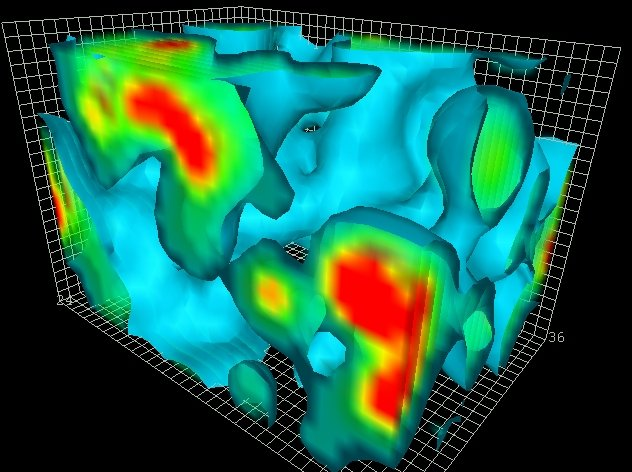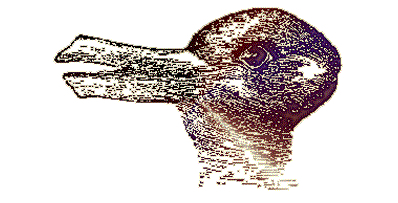# David Skinner: Lectures on Theoretical Physics## Mathematical Methods

An introduction to the Laplace, heat and wave equations, using Fourier Series and Transforms and the method of characteristics. Aimed at second year undergraduates.## Principles of Quantum Mechanics

A second course on quantum mechanics, using Dirac's notation. Introduces Hilbert spaces, unitary representations of Lie groups, and perturbation theory. Aimed at third year undergraduates.## Quantum Field Theory

A second course in Quantum Field Theory, aimed at Part III Masters students. It covers the Wilsonian renormalization group, Effective Field Theory, and non-Abelian gauge theories.## Supersymmetry

An introduction to Supersymmetry, aimed at Part III Masters students. It focuses on mathematical applications of supersymmetric theories, such as index theorems, localization, quantum cohomology and Donaldson-Witten theory.